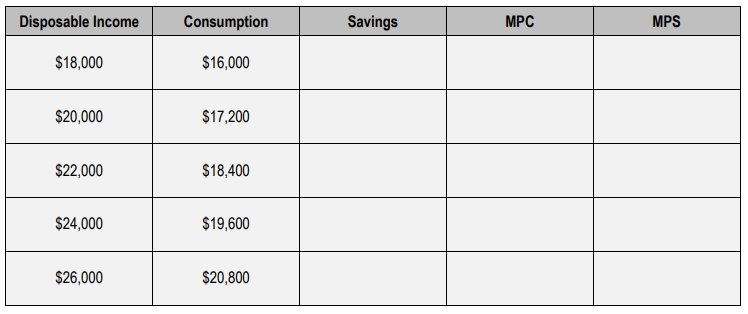Clutch Prep is now a part of Pearson
Ch. 15 - Income and ConsumptionWorksheetSee all chapters

# The Consumption Function

See all sections
Sections
The Consumption Function
The Saving Function
Determinants of Consumption and Saving
Average Propensity to Consume and Save
Multiplier Effect of Investment Spending

Concept #1: Consumption Function (Consumption Schedule)

Concept #2: Marginal Propensity to Consume and Save

Concept #3: 1 = MPC + MPS

Concept #4: Equation of the Consumption Function

Practice: Calculate the Marginal Propensity to Consume and the Marginal Propensity to Save using the following table:Practice: If the Keynesian consumption function is C = 10 + 0.8 Yd then, if disposable income is $1000, what is amount of total consumption? Practice: If the Keynesian consumption function is C = 10 + 0.8 Yd then, when disposable income is$1000, what is the marginal propensity to consume?

Practice: An increase in the marginal propensity to consume will: